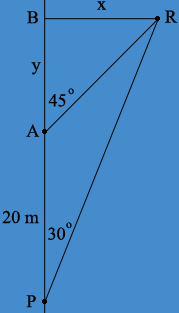SEARCH HOMEMath Central Quandaries & QueriesQuestion from Amy, a student: Susan notices an unusual rock formation at an angle of 30 degrees to the right of her direction of travel as she is white water rafting. as she continues in a relatively straight path for 20 m, the rock formation appears to be at 45 degrees to the right. she continues until the rock formation is directly to her right. how far is she from he rock formation?Hi Amy,

In my mind the key here is a good, labeled, diagram. Here is mine.Susan starts at $P$, moves t $A$ which is 20 m away, and the to $B$ from which the rock formation $R$ is directly to her right. This means that the angle $RBA$ is a right angle. Hence triangles $RBA$ and $RBP$ are right triangles.

Can you complete the problem now? Write back if you need more assistance.
PennyMath Central is supported by the University of Regina and The Pacific Institute for the Mathematical Sciences.